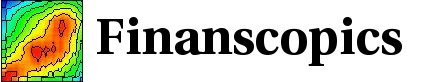Base information Indexes

# Purposes

This site originates from the following question: within the dozens of processes that attempt to capture the empirical properties of financial time series, which ones can or cannot describe the various stylized facts? Another way to express this question is: what are the properties of a given time series, being a process or empirical data. The purpose of this site is to display properties of financial time series in a uniform way, in order to allow for an easy comparison. In this way, the merits and drawbacks of each process can be compared easily, either with empirical data or with other processes. For the processes, this allows to relate the form of the mathematical equations to the statistical properties of the generated time series.

For example, the empirical time series exhibit "long memory" for the volatility, namely the lagged volatility correlation decays slowly. The GARCH(1,1) process has an exponential memory (created by the multiplicative structure of the equations), and therefore it can only reproduce approximately this stylized fact. On the other hand, the GARCH(1,1) process has other interesting properties, for example some analytical tractability.

For a given process, the quantitative value for a properties are depending on the process parameters. By adjusting parameters, one selected property could be reproduced better, within some limits. For example, the value of the lagged correlation for the GARCH(1,1) process can be adjusted to be close to the empirical values in a range of time lags. Yet, the exponential decay will never reproduce the empirical slow decay, but only provides for a good approximation within the selected time lags. For this reason, the focus is on the qualitative feature of the processes, and the process parameters are chosen so as to give a good overall approximation of the empirical properties.

The core of this site is a set of graphs for each time series showing the various salient properties according to a broad set of statistics. The computed quantities and graphs are identical in order to allow for a uniform comparison of the statistical properties. For each time series, a set of web pages display the graphs. For example, one page shows the lagged correlation of the volatility with different representation (linear-linear, linear-log, log-log), in this particular example for CHF/USD.

Roughly, an index is given for each time series, allowing to access the pages for the various properties. On these property pages, the graphs display a set of related statistics (as in the previous example about the volatility lagged correlation). An explanation for each figure is given on a set of similar pages. The indexes per time series are accessed from the main link index. Other ways to select time series and/or graphs are presented in the home page, and can be accessed through the links given on the upper right for each pages.